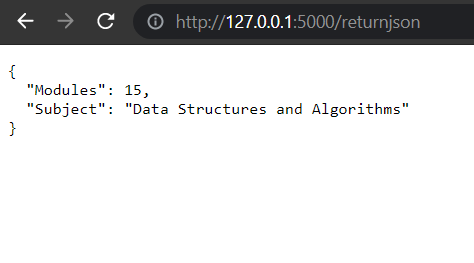GeeksforGeeks App
Open AppBrowser
Continue

# How to return a JSON response form a Flask API ?

Flask is one of the most widely used python micro-frameworks to design a REST API. In this article, we are going to learn how to create a simple REST API that returns a simple JSON object, with the help of a flask.

Prerequisites: Introduction to REST API

What is a REST API?

REST stands for Representational State Transfer and is an architectural style used in modern web development. It defines a set or rules/constraints for a web application to send and receive data. In this article, we will build a REST API in Python using the Flask framework. Flask is a popular micro framework for building web applications.

Approaches: We are going to write a simple flask API that returns a JSON response using two approaches:

Libraries Required:

• Install the python Flask library using the following command:
`pip install Flask`
• Install the flask-restful library using the following command:
`pip install Flask-RESTful`

Approach 1: Using Flask jsonify object – In this approach, we are going to return a JSON response using the flask jsonify method. We are not going to use the flask-restful library in this method.

• Create a new python file named ‘main.py’.
• Register the web app into an app variable using the following syntax.
`app = Flask(__name__)`
• Create a new function named ‘ReturnJSON’. This function is going to return the sample JSON response.
• Route the ‘ReturnJSON’ function to your desired URL using the following syntax.
```@app.route('/path_of_the_response', methods = ['GET'])
def ReturnJSON():
pass```
• Inside the ‘ReturnJSON’ function if the request method is ‘GET’ then create a python dictionary with the two elements message.
• Jsonify the python dictionary and return it.
• Build the flask application using the following command.
```if __name__=='__main__':
app.run(debug=True)```
• Run the ‘main.py’ file in the terminal or the IDE.

Code:

## Python3

 `from` `flask ``import` `Flask,jsonify,request`` ` `app ``=`   `Flask(__name__)`` ` `@app``.route(``'/returnjson'``, methods ``=` `[``'GET'``])``def` `ReturnJSON():``    ``if``(request.method ``=``=` `'GET'``):``        ``data ``=` `{``            ``"Modules"` `: ``15``,``            ``"Subject"` `: ``"Data Structures and Algorithms"``,``        ``}`` ` `        ``return` `jsonify(data)`` ` `if` `__name__``=``=``'__main__'``:``    ``app.run(debug``=``True``)`

Output:Approach 2: Using the flask_restful library with Flask – In this approach, we are going to create a simple JSON response with the help of the flask-restful library. The steps are discussed below:

• Create a new python file named ‘main.py’.
• Import API and Resource from the ‘flask_restful’ library.
• Register the web app into an app variable using the following syntax.
`app = Flask(__name__)`
• Register the app variable as an API object using the API method of the ‘flask_restful’  library.
`api = Api(app)`
• Create a resource class named ‘ReturnJSON’.
• Inside the resource, the class creates a ‘get’ method.
• Return a dictionary with the simple JSON response from the ‘get’ method.
• Add the resource class to the API using the add_resource method.
• Build the flask application using the following command.
```if __name__=='__main__':
app.run(debug=True)```
• Run the ‘main.py’ file in the terminal or the IDE.

Code:

## Python3

 `from` `flask ``import` `Flask``from` `flask_restful ``import` `Api, Resource`` ` `app ``=`   `Flask(__name__)`` ` `api ``=`   `Api(app)`` ` `class` `returnjson(Resource):``    ``def` `get(``self``):``        ``data``=``{``            ``"Modules"``: ``15``, ``            ``"Subject"``: ``"Data Structures and Algorithms"``        ``}``        ``return` `data`` ` `api.add_resource(returnjson,``'/returnjson'``)`` ` ` ` `if` `__name__``=``=``'__main__'``:``    ``app.run(debug``=``True``)`

Output:My Personal Notes arrow_drop_up
Related Tutorials